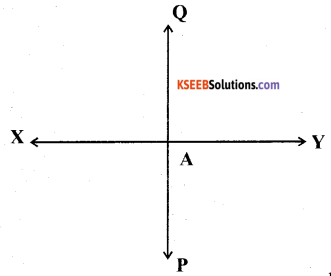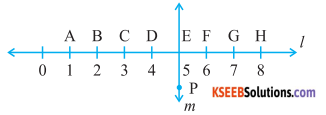# KSEEB Solutions for Class 6 Maths Chapter 5 Understanding Elementary Shapes Ex 5.5

Students can Download Chapter 5 Understanding Elementary Shapes Ex 5.5 Questions and Answers, Notes Pdf, KSEEB Solutions for Class 6 Maths helps you to revise the complete Karnataka State Board Syllabus and score more marks in your examinations.

## Karnataka State Syllabus Class 6 Maths Chapter 5 Understanding Elementary Shapes Ex 5.5

Question 1.
Which of the following are models for perpendicular lines:
Solution:
a) The adjacent angles of a table top
The adjacent edge of a table top are perpendicular to each other.
b) The line of a railway track
The lines of a railway track are parallel to each other.
c) The line segments forming the letter ‘L’
The line segments forming the letter ‘L’ are perpendicular to each other.
d) The letter V.
The sides of letter V are inclined at some a cute angle on each other
Hence (a) and (c) are the models for perpendicular lines.Question 2.
Let $$\overline{\mathbf{P Q}}$$ be the perpendicular to the line segment $$\overline{\mathbf{X Y}}$$. Let $$\overline{\mathbf{P Q}}$$ and $$\overline{\mathbf{X Y}}$$ intersect in the point A. What is the measure of ∠PAY?
Solution:From the figure if c be easily observed that the measure of ∠PAY is 90°

Question 3.
There are two set-squares in your box. What are the measures of the angles that are formed at their corners? Do they have any angle measure that is common?
Solution:
On has a measure of 90°, 45°, 45° other has a measure of 90°, 30°, 60° Therefore, the angle of 90° measure is common between them.Question 4.
Study the diagram. The line l is perpendicular to line m
Solution:
a) Is CE = EG?Yes, As CE = EG = 2 units

b) Does PE bisect CG?
Yes, PE bisects CG since CE = EG

c) Identify any two line segments for which PE is the perpendicular bisector.
DF and BHd) Are these true?
i) AC < EG
True, As length of AC and FG are of 2 units and 1 unit respectively

ii) CD = GH
True, As both have 1 unit length

iii) BC < EH
True, As the length of BC and EH are of 1 unit and 3 units respectively.